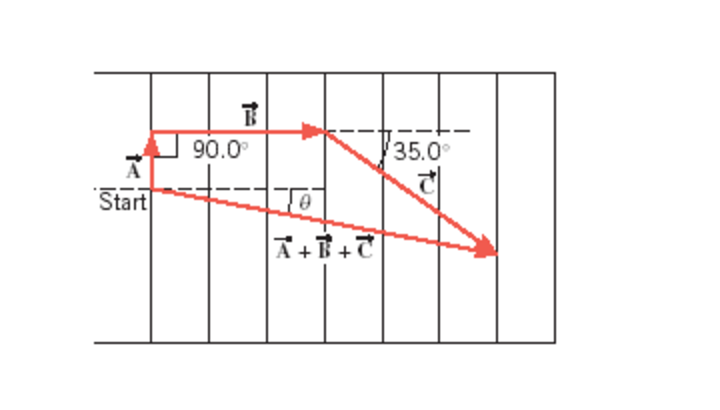# A football player runs the pattern given in the drawing

A football player runs the pattern given in the drawing by the three displacement vectors A, B, and C. The magnitudes of these vectors are A = 4.00m, B = 16.0m, C = 19.0m. Using the component method, find the:

a.) Magnitude

b.) Direction theta of the resultant vector A + B + C. Take theta to be a positive angle.rn